# Points, lines, line segments, and rays#### Everything You Need in One Place

Homework problems? Exam preparation? Trying to grasp a concept or just brushing up the basics? Our extensive help & practice library have got you covered.#### Learn and Practice With Ease

Our proven video lessons ease you through problems quickly, and you get tonnes of friendly practice on questions that trip students up on tests and finals.#### Instant and Unlimited Help

Our personalized learning platform enables you to instantly find the exact walkthrough to your specific type of question. Activate unlimited help now!

0/5
##### Intros
###### Lessons
1. Introduction to Points, Lines, Line Segments and Rays:
2. Introduction to basic figures and points
3. What are lines?
4. What are line segments?
5. What are rays?
6. Review of points, lines, line segments and rays
0/18
##### Examples
###### Lessons
1. Identifying lines, line segments, and rays
Name the figure that is made by points $A$ and $B$ as either: a line, a line segment, or a ray.
1.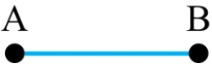2.3.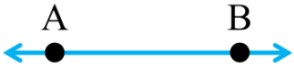4.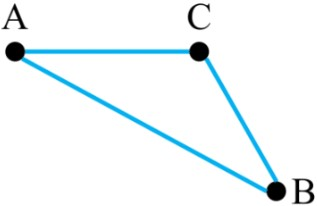5.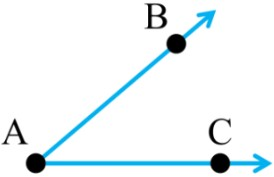2. Proper notation for lines, line segments and rays
Use proper notation (with letters and symbols) to name the figure
1.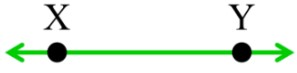2.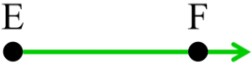3.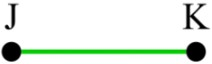3. Drawing lines, line segments and rays
Draw the figure:
1. ray $\overrightarrow{MN}$
2. line segment $\overline{QR}$
3. line $\overleftrightarrow{GH}$
4. Combinations of lines, line segments and rays
Name all the lines, line segments, and/or rays in the picture. Use proper notation.
1.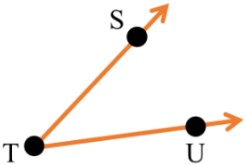2.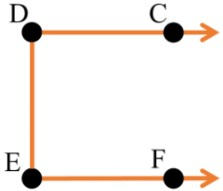3.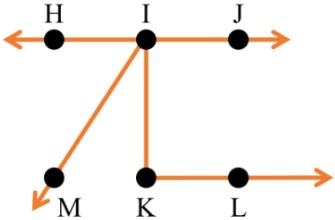5. Intersecting lines, line segments and rays
Connect the dots to draw the figures as indicated. Will the figure intersect? Yes or No.
1. $\overrightarrow{XW}$ and $\overleftrightarrow{YZ}$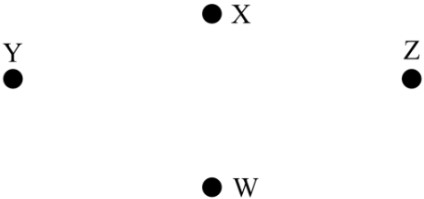2. $\overline{AB}$ and $\overrightarrow{DC}$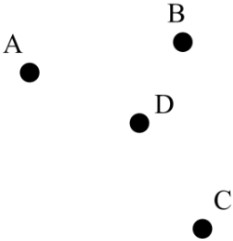3. $\overline{NO}$ and $\overrightarrow{PQ}$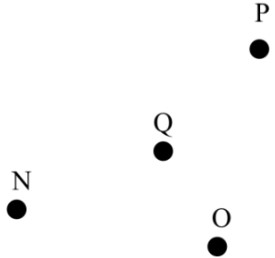4. $\overline{RS}$ and $\overleftrightarrow{TU}$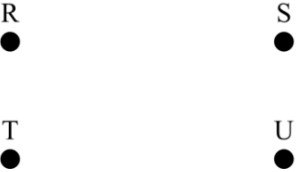0%
##### Practice
###### Topic Notes

In this lesson, we will learn:

Notes:

• Points, Lines, Line Segments and Rays are basic figures in math that can be used to construct more complicated figures (i.e. angles, shapes, parallel/perpendicular lines, etc.)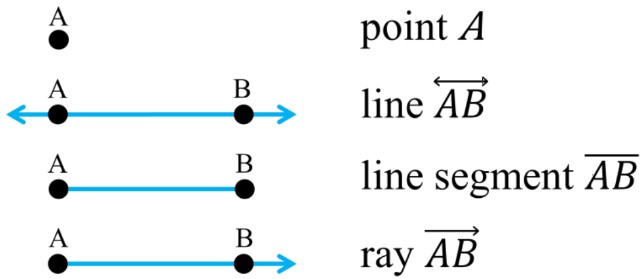• A point is a dot with position but no size nor shape (undefined) $\,$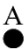• There are an infinite number of points in figures (i.e. you can draw many points between endpoints in a line segment) $\,$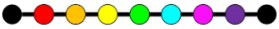• The proper notation of a point is just to write the named letter (ex. point $A$)

• A line has no beginning/end; an ongoing straight path of points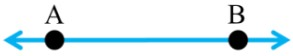• The arrows on both sides mean that it can extend infinitely in that direction
• The proper notation of a line is to write the letters of two points in the figure and draw a double-sided arrow above the letters (ex. line $\overleftrightarrow{AB}$)

• A line segment is a part of a line with two finite endpoints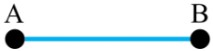• A line has arrows on both ends whereas the line segment has endpoints on both ends; the line continues forever, where the line segment ends where it is drawn
• The proper notation of a line segment is to write the letters of the two endpoints and draw a bar above the letters (ex. line segment $\overline{AB}$)

• A ray starts at one endpoint and extends in one direction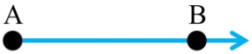• One end of the ray has a point, whereas the other end of the ray has an arrow
• The proper notation of a ray is to write the letters of two points in the figure (starting with the letter of the endpoint) and draw a one-sided arrow above the letters (ex. ray $\overrightarrow{AB}$ )